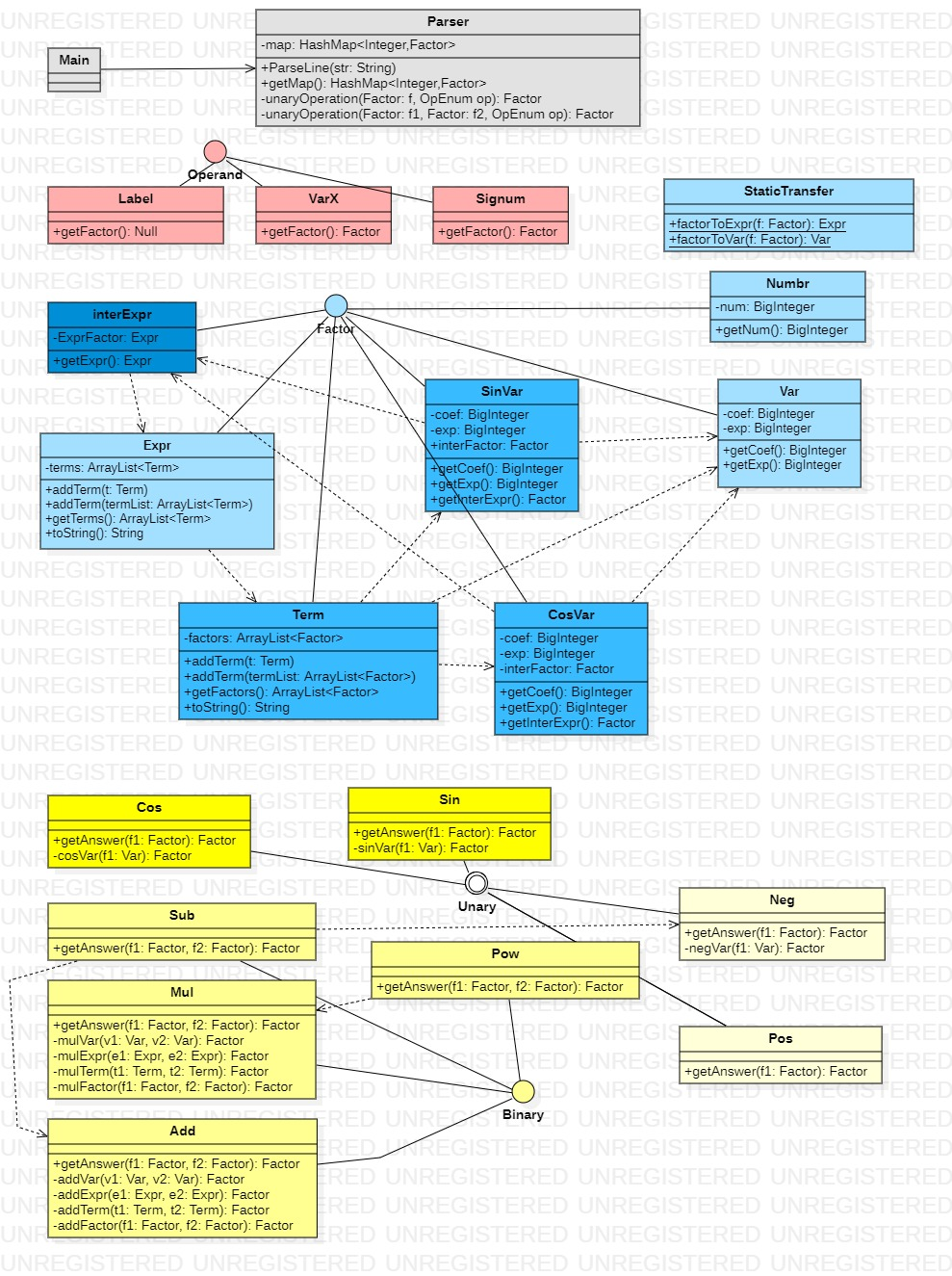# OO第一单元总结

## 一、度量分析

### 1.1 类图分析

• 第二次作业在第一次作业的基础上增加了两类因子sin因子 cos因子和两个操作符sincos
• 第三次作业sin因子cos因子内部储存的因子自变量除了是x以外，还可以是表达式。

Parser类为主要的功能类，由Main类关联控制其生命流程。该类内部属性map:HashMap<Integer,Factor>存有所有标签值和其对应的表达式。

Factor接口用来实现储存表达式、因子、项的类；在第一次作业中，项可以通过系数+x指数的方式实现，

StaticTransfer静态类，提供Factor的实现类转化为项或表达式的方法，便于运算器计算。

Operand接口为操作数，操作数可以是标签，可以是变量x，也可以是带符号整数，分别由三个类 Label, VarX, Signum 实现；

BinaryUnary 接口为双目和单目运算器接口，具体的实现类负责完成操作符代表的运算来处理操作数。

#### 1.1.1 第一次作业的类图：#### 1.1.2 第二次作业的类图：#### 1.1.3 第三次作业的类图：### 1.2 复杂度度量

@Override
public String toString() {
if (coef.equals(BigInteger.ZERO)) {
return "0";
} else {
if (exp.equals(BigInteger.ZERO)) {
return this.coef.toString();
} else {
if (coef.equals(BigInteger.ONE)) {
if (exp.equals(BigInteger.ONE)) {
return "x";
} else {
return "x**" + exp.toString();
}
} else if (coef.equals(BigInteger.ONE.negate())) {
if (exp.equals(BigInteger.ONE)) {
return "-x";
} else {
return "-x**" + exp.toString();
}
} else {
if (exp.equals(BigInteger.ONE)) {
return coef.toString() + "*x";
} else {
return coef.toString() + "*x**" + exp.toString();
}
}
}
}
}

method ev(G) iv(G) v(G)
expr.Var.toString() 8.0 8.0 8.0
calculator.Mul.mulFactor(Factor,Factor) 3.0 3.0 4.0
calculator.Neg.getAnswer(Factor) 3.0 4.0 4.0
calculator.Pow.getAnswer(Factor,Factor) 3.0 3.0 3.0
calculator.Pow.powInt(Factor,BigInteger) 3.0 4.0 4.0
calculator.Mul.getAnswer(Factor,Factor) 2.0 2.0 4.0
option.Operator.Operator(String) 2.0 2.0 8.0
Main.main(String[]) 1.0 3.0 3.0
Parser.Parser() 1.0 1.0 1.0
Parser.binaryOperation(Factor,Factor,OpEnum) 1.0 7.0 7.0
Parser.getMap() 1.0 1.0 1.0
Parser.parseLine(String) 1.0 3.0 5.0
Parser.strToFactor(String) 1.0 3.0 3.0
Parser.unaryOperation(Factor,OpEnum) 1.0 3.0 3.0
calculator.Mul.mulExpr(Expr,Expr) 1.0 3.0 3.0
calculator.Mul.mulVar(Var,Var) 1.0 1.0 1.0
calculator.Neg.negVar(Var) 1.0 1.0 1.0
calculator.Pos.getAnswer(Factor) 1.0 1.0 1.0
calculator.StaticTransfer.factorToExpr(Factor) 1.0 3.0 5.0
calculator.StaticTransfer.factorToVar(Factor) 1.0 2.0 3.0
calculator.Sub.getAnswer(Factor,Factor) 1.0 1.0 1.0
expr.Expr.Expr() 1.0 1.0 1.0
expr.Expr.Expr(ArrayList) 1.0 1.0 1.0
expr.Expr.addTerm(ArrayList) 1.0 1.0 1.0
expr.Expr.addTerm(Var) 1.0 1.0 1.0
expr.Expr.getTerms() 1.0 1.0 1.0
expr.Expr.toString() 1.0 4.0 4.0
expr.Numbr.Numbr(BigInteger) 1.0 1.0 1.0
expr.Numbr.getNum() 1.0 1.0 1.0
expr.Numbr.toString() 1.0 1.0 1.0
expr.Var.Var() 1.0 1.0 1.0
expr.Var.Var(BigInteger,BigInteger) 1.0 1.0 1.0
expr.Var.getCoef() 1.0 1.0 1.0
expr.Var.getExp() 1.0 1.0 1.0
option.Label.Label(String) 1.0 1.0 1.0
option.Label.Label(int) 1.0 1.0 1.0
option.Label.getFactor() 1.0 1.0 1.0
option.Label.getKey() 1.0 1.0 1.0
option.Operator.getOp() 1.0 1.0 1.0
option.SigNum.SigNum(BigInteger) 1.0 1.0 1.0
option.SigNum.SigNum(String) 1.0 1.0 1.0
option.SigNum.getFactor() 1.0 1.0 1.0
option.VarX.getFactor() 1.0 1.0 1.0
Total 68.0 92.0 109.0
Average 1.446808510638298 1.9574468085106382 2.3191489361702127

## 二、Bug分析

### 2.1 自身bug分析

class XxxTest { // xxx classname
Xxx x;

@BeforeEach
void setUp() {
ArrayList<parameterType> list1 = new ArrayList<>();
ArrayList<parameterType> list2 = new ArrayList<>();
parameterType item11 = ...;
parameterType item12 = ...;
...
parameterType item21 = ...;
parameterType item22 = ...;
...
...
...
}

@Test
void xx() { // xx methodname
int cnt = 0;
for (parameterType i : List1){
for (parameterType j : List2){
System.out.println("cnt: " + cnt + " | " + i.toString() + " " + j.toString());
cnt++;
parameterType k = Xxx.xx(i, j);// ans
System.out.println("ansTypeis: " + k.getClass().getTypeName() + " | " + k.toString());
}
}
}
}



## 四、心得体会

posted @ 2022-03-26 09:11    阅读(22)  评论(0编辑  收藏  举报